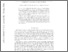REAL

# Rank, combinatorial cost, and homology torsion growth in higher rank lattices

Abért, Miklós and Gelander, T. and Nikolov, N. (2017) Rank, combinatorial cost, and homology torsion growth in higher rank lattices. DUKE MATHEMATICAL JOURNAL, 166 (15). pp. 2925-2964. ISSN 0012-7094Preview
Text
1509.01711v2.pdf

Download (363kB) | Preview

## Abstract

We investigate the rank gradient and growth of torsion in homology in residually finite groups. As a tool, we introduce a new complexity notion for generating sets, using measured groupoids and combinatorial cost. As an application we prove the vanishing of the above invariants for Farber sequences of subgroups of right-angled groups. A group is right angled if it can be generated by a sequence of elements of infinite order such that any two consecutive elements commute. Most nonuniform lattices in higher rank simple Lie groups are right angled. We provide the first examples of uniform (cocompact) right-angled arithmetic groups in SL(n,ℝ), n ≥ 3, and SO(p, q) for some values of p, q. This is a class of lattices for which the congruence subgroup property is not known in general. By using rigidity theory and the notion of invariant random subgroups it follows that both the rank gradient and the homology torsion growth vanish for an arbitrary sequence of subgroups in any right-angled lattice in a higher rank simple Lie group. © 2017.

Item Type: Article Q Science / természettudomány > QA Mathematics / matematika MTMT SWORD MTMT SWORD 12 Feb 2018 07:37 12 Feb 2018 07:37 http://real.mtak.hu/id/eprint/74284

### Actions (login required)Edit Item